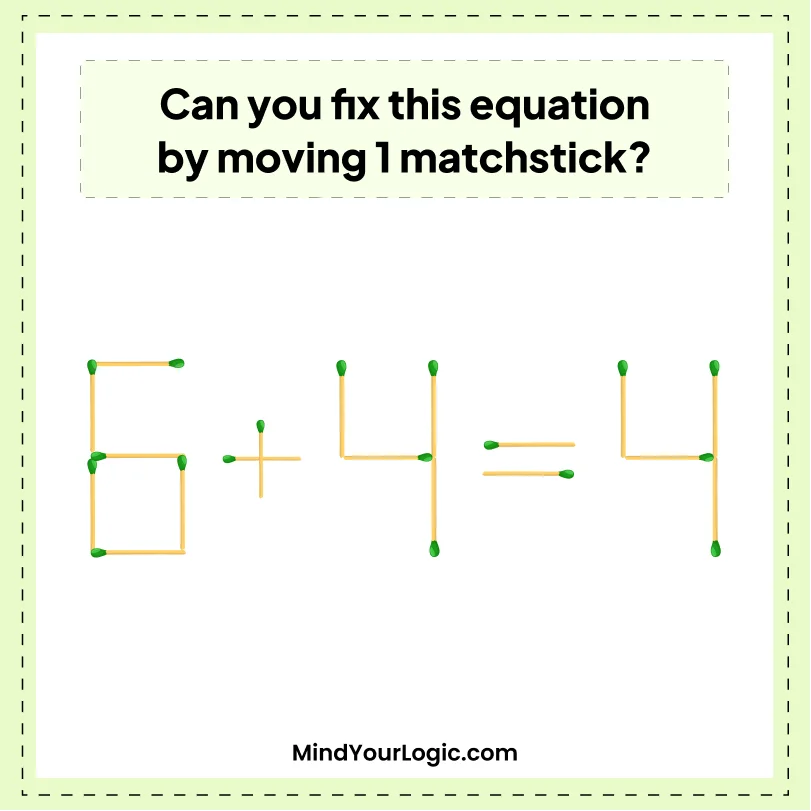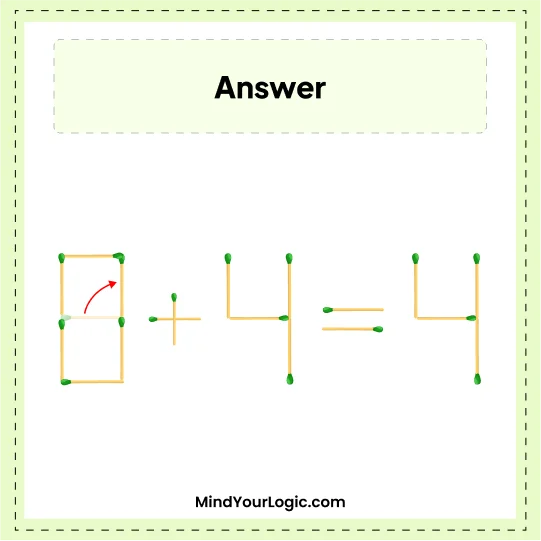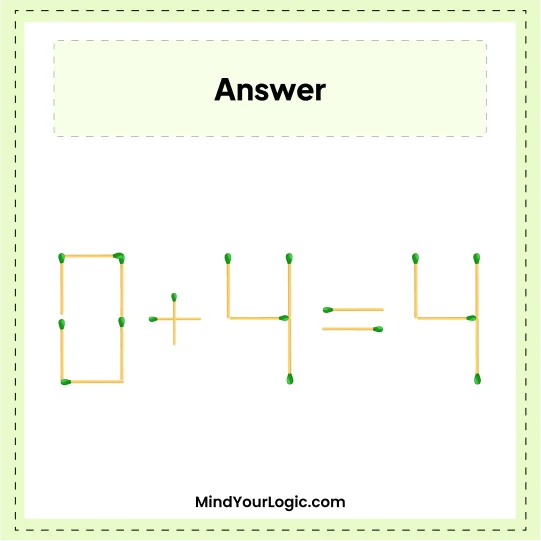# 6+4=4 - Matchstick Puzzle

###### 32.Matchstick Puzzles
`Test your IQ, try to solve this equation by moving only one matchsticks. `•

Explanation :

` Move one stick from 6 and place it in a standing position. Equation will become like 0 + 4 = 4. `